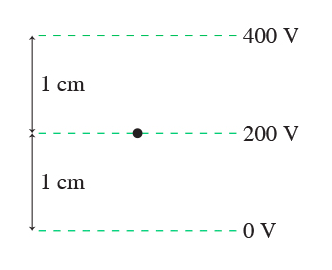# Problem: What is the magnitude of the electric field at the dot? Express your answer to one significant figure and include the appropriate units.

###### FREE Expert Solution

Electric field:

$\overline{){\mathbf{E}}{\mathbf{=}}\frac{\mathbf{∆}\mathbf{V}}{\mathbf{d}}}$

The distance, d between the surfaces is 1 cm.

89% (175 ratings)###### Problem Details

What is the magnitude of the electric field at the dot?Express your answer to one significant figure and include the appropriate units.